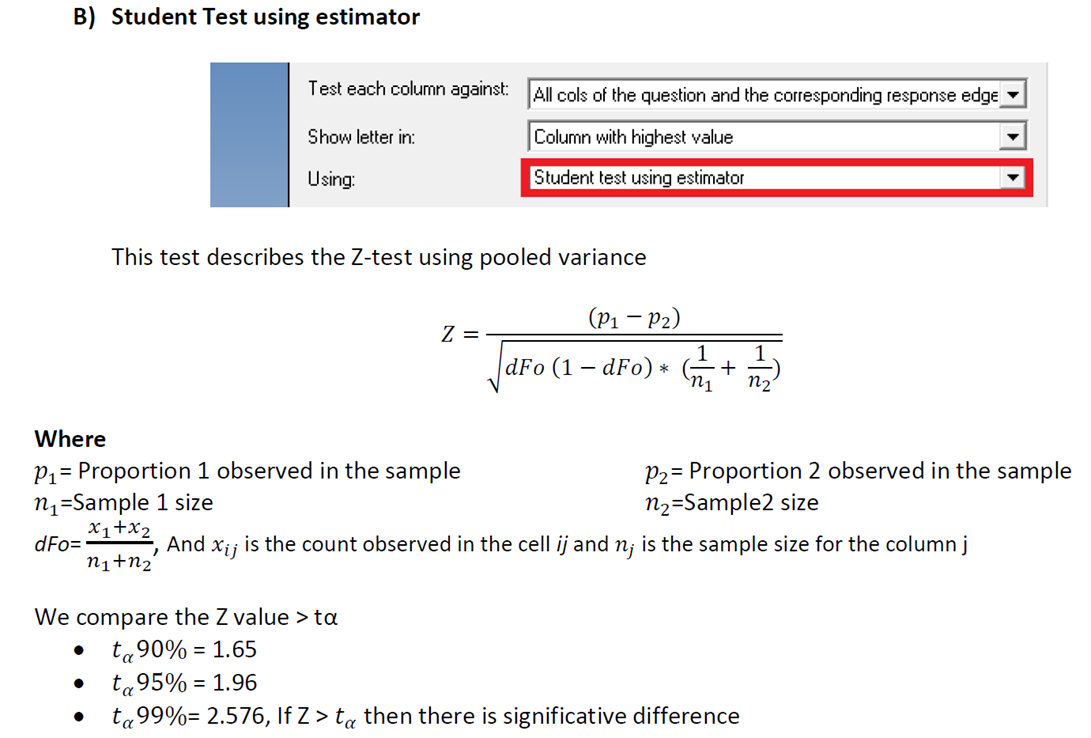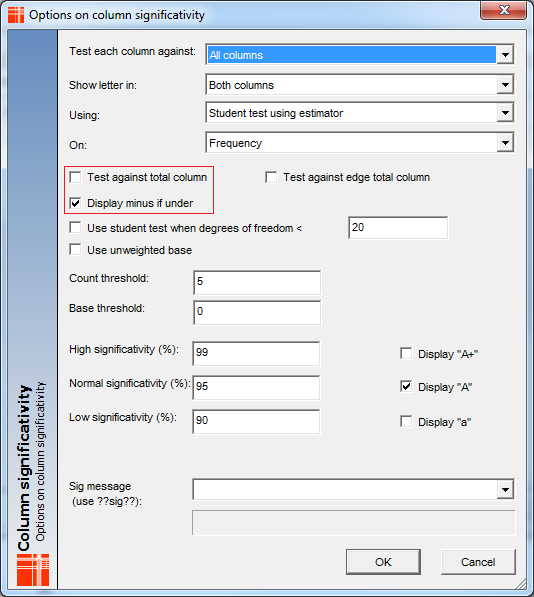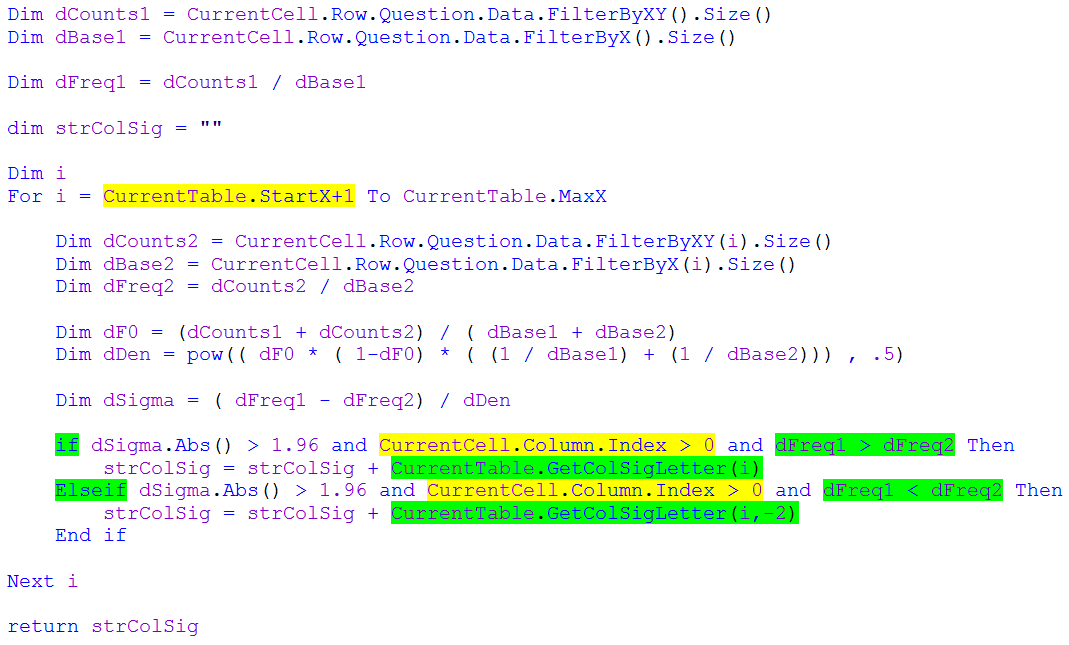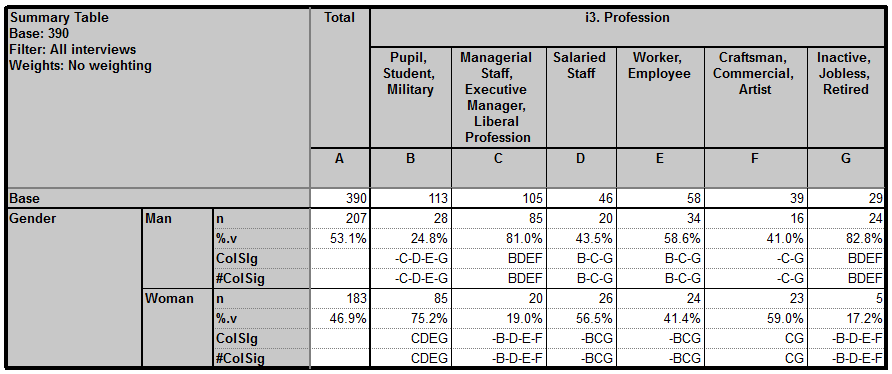Scroll

# Programming Col Sig (proportions): Part 2 - (Student Test using estimator)

Follow
 Summary This article demonstrates how to script the formula for Student Test using estimator on closed questions. Formula and syntax are shown along with tips on how to mirror particular settings in the pre-set col sig calculation. Applies to Askia Analyse. Written for Researchers, Developers, Data Processors. Keywords Table arithmetic, Forloops, Coordinates, data, Calculation arithmetic, Bespoke calculation, Filtered, Multiplication table, Classical Student Test, Estimator, Sigma2, Z-test, Pooled variance, Confidence interval, 95%, FilterBy, GetColSigLetter.

The .qes file and portfolio containing the examples discussed is attached: Table Arithmetic Examples 11 & 12.rar.

Student Test using estimator (Sigma2)

In this demonstration I will create a table which shows the pre-set colsig calculation side by side with the programmed calculation for the same test. First, let's review the formula:The pre-set calculation looks like this in Analyse:

Calc: ColSigIn the syntax below I show how to achieve these settings. Notice that 'Test against total column' is not ticked - this setting is dealt with by the parts of the syntax highlighted in yellow.

Also, 'Display minus if under' is ticked. This dealt with by the parts of the syntax highlighted in green.

Calc: #ColSigYou can see the result of this by running tab definition: Sigma2 in the example portfolio:Was this article helpful?
0 out of 0 found this helpful
Have more questions? Submit a request